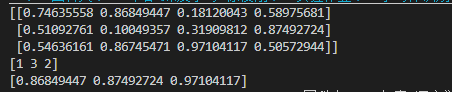• 现在有一个二维数组test_y，我想要获取每一行的最大值，以及最大值所在索引值 代码： 随机生成一个3行4列的数组，利用np.argmax（）函数获取最大值所在索引，amax（）获取最大值的值。 import numpy as np test...
任务描述：
现在有一个二维数组test_y，我想要获取每一行的最大值，以及最大值所在索引值
代码：
随机生成一个3行4列的数组，利用np.argmax（）函数获取最大值所在索引，amax（）获取最大值的值。
import numpy as np

test_y = np.random.rand(3,4)
idx = np.argmax(test_y, axis=1)
pro = np.amax(test_y, axis=1)

print(idx)
print(pro)
运行结果展开全文python
• 主要介绍了JavaScript如何获取数组最大值和最小值，需要的朋友可以参考下
• 主要介绍了PHP获取数组最大值下标的方法,涉及php中max及array_search等函数操作数组的相关技巧,需要的朋友可以参考下
• ES6获取数组最大值
const a = [1, 2, 3, 4, 5, 6];
const max = Math.max(...a);
console.log(max); // 6

展开全文javascript
• 主要介绍了JS获取数组最大值、最小值及长度的方法,涉及JavaScript遍历数组及length属性的相关使用技巧,非常简洁实用,需要的朋友可以参考下
• ## Java获取数组最大值

万次阅读 多人点赞 2018-07-31 09:31:45
简述 方法其实有很多种的。... System.out.println("最大值: " + max); } } 实例三： import java.util.Arrays public static int MAX(int[] arr) { return Arrays.stream(arr).max().getAsInt(); }
简述
方法其实有很多种的。我先放一些，之后有再遇到对应代码再放上来。
最简单的当然是一个个找进行对比的方法啦~ 当然还是有一些有趣的操作的
实例一：
import java.util.Arrays;

public static int MAX(int[] arr) {
Arrays.sort(arr);
return arr[arr.length-1];
}


就是先排序再来得到结果
实例二 这个是菜鸟教程上的一份代码
import java.util.Arrays;
import java.util.Collections;

public class Main {
public static void main(String[] args) {
Integer[] numbers = { 8, 2, 7, 1, 4, 9, 5};
int min = (int) Collections.min(Arrays.asList(numbers));
int max = (int) Collections.max(Arrays.asList(numbers));
System.out.println("最小值: " + min);
System.out.println("最大值: " + max);
}
}

实例三：
import java.util.Arrays
public static int MAX(int[] arr) {
return Arrays.stream(arr).max().getAsInt();
}展开全文java
• javaScript获取数组最大值和最小值 获取数组的最大值和最小值是常用需求，实现的方式也多种多样，下面介绍几个获取数组最大值最小值的方法。 1.遍历法 例如求最大值，假设数组第一个就是最大值，然后遍历数组，拿这...
javaScript获取数组最大值和最小值
获取数组的最大值和最小值是常用需求，实现的方式也多种多样，下面介绍几个获取数组最大值最小值的方法。
1.遍历法
例如求最大值，假设数组第一个就是最大值，然后遍历数组，拿这个最大值和后面的元素逐一比较，把假设的值进行替换。最后得到的结果就是我们想要的。
// 获取最大值：
var arr = [2,23,12,124,82];
var max = arr;

for(var i = 1; i < arr.length; i++) {
var cur = arr[i];
cur > max ? max = cur : null
}

console.log(max); // 124

最小值同理。
// 获取最小值：
var arr = [2,23,12,124,82];
var min = arr;

for(var i = 1; i < arr.length; i++) {
var cur = arr[i];
cur < min ? min = cur : null
}
console.log(min)  // 2

2.排序法
把数组升序排序，第一项就是最小值，最后一项就是最大值；把数组降序排序，第一项就是最大值，最后一项就是最小值。
var arr = [2,23,12,124,82];

arr.sort(function (a, b) {
return a-b;
}); // 升序排序 return b-a 为降序排序
var min = arr;  // 2
var max = arr[arr.length - 1];  //124

3.Math的max/min方法
var arr = [2,23,12,124,82];

var max = Math.max.apply(null, arr);
var min = Math.min.apply(null, arr);

console.log(max, min) // 124,2

4.ES6的扩展运算符
//最大值
var arr = [2,23,12,124,82];
console.log(arr.Math(...arr)); // 124

5.eval
本质上还是使用Math的max/min方法
var arr = [2,23,12,124,82];
var max = eval("Math.max(" + arr + ")");
console.log(max); //124

展开全文javascript
• package zzx.base.maxmin;... * @return 定义一个获取数组最大值的静态方法 */ public static int getMax(int[] arr) { // 定义一个参照物 int max = arr; //遍历数组 for (int i =...
• JavaScript获取数组最大值和最小值 //获取数组最大值 function findMax() { var arr = [3,4,76,2,54,23,78,90,89,99,234,123,456,23,76,89,90]; var max = Array.max(arr); document.getElementById("max...JavaScript 最小值
• Java获取数组最大值和最小值 声明2个变量，取数组任意两个值进行赋值 遍历数组，分别与这两值比较，比当前最大值大时，把它赋值给最大值，比当前最小值小时，把它赋值给最小值。 public class GetMaxMin ...java 算法 编程语言
• * 获取数组最大值和下标 * @param x * @param y * @return */ private double peakCenterResult(double[] x, double[] y) { double peakCenter; ArrayList&lt;Double&gt; darraylist=new ...
• 获取数组最大值和最小值 废话少说，直接上代码，说白了就是基础自己学，方法可以参考 import java.util.Arrays; public class Test_获取最大值和最小值 { public static void main(String[] args) { //定义一个...java
• js获取数组最大值最小值max,min 点击查看文章内容 数组排序，获取第一个和最后一个为最小值最大值;此方法通用 var arr=[2,3,5,6]; var sortArr=arr.sort(function(a,b){return a-b;}); var min=sortArr; var ...Math.min
• 获取数组最大值和最小值操作 利用Java的Math类的random()方法，编写函数得到0到n之间的随机数，n是参数。 并找出产生50个这样的随机数中最大的、最小的数，并统计其中&gt;=60的有多少个。 提示：使用 int num=...
• ## java获取数组最大值

万次阅读 2013-07-31 00:15:48
* 获取数组最大值  * 思路：  * 1、获取最值需要进行比较，每一次比较都会有一个较大的值，因为该值不确定通过一个变量进行临存.  * 2、让数组中的每一个元素都和这个变量中的值进行比较。如果大于了变量中...
• js获取数组最大值的四种方式 var arr = [7,2,0,-3,5]; 1 1.apply()应用某一对象的一个方法，用另一个对象替换当前对象 var max = Math.max.apply(null,arr); console.log(max) 由于max()里面参数不能为数组，...
• ## php获取数组最大值

万次阅读 2018-10-10 09:50:04
php获取一维数组里的最大值和最小值的方法。 php获取一维数组里面的最大值： &lt;?php $a=array('1','3','55','99'); echo max($a); php获取一维数组里面的最小值： &lt;?php \$a=array('1','3','55','...
• 1 获取数组最大值和最小值 */ class ArryTest { /* 获取数组最大值 思路： 1，获取最值需要比较，每一次都会有一个较大的值。因为该值不确定， 通过一个变量进行临时存储 2，让数组中的每一个元素都和...
• 代码示例： import numpy as np ... 获取所有数据最大值 result = np.max(score) print(result) #打印65 # 2. 获取某一个轴上的数据最大值 result = np.max(score,axis=0) print(result) #打印[62 65] ...numpy
• // 获取数组元素的最大值和最小值 int main(void){ int arr[] = {1,2,3,5,67,8,9,33}; int min,max; compute_m(arr,sizeof(arr)/sizeof(int),&min,&max); printf("result: min = %d , max = %d \nc语言 pointer...